+
Calculating Standard Deviation and Variance
1 Tutorials that teach Calculating Standard Deviation and Variance

# Calculating Standard Deviation and Variance

Author: Sophia Tutorial
##### Description:

Calculate standard deviation.

(more)

Sophia’s self-paced online courses are a great way to save time and money as you earn credits eligible for transfer to many different colleges and universities.*

No credit card required

37 Sophia partners guarantee credit transfer.

299 Institutions have accepted or given pre-approval for credit transfer.

* The American Council on Education's College Credit Recommendation Service (ACE Credit®) has evaluated and recommended college credit for 32 of Sophia’s online courses. Many different colleges and universities consider ACE CREDIT recommendations in determining the applicability to their course and degree programs.

Tutorial
what's covered
This lesson discusses representing how data can vary. You will be able to identify the symbols for sample variance and sample standard deviation. You will also learn the correct formula for and be able to determine the sum of squares of samples. This lesson covers:
1. Variability
2. Standard Deviation
3. Variance

## 1. Variability

In addition to finding the center of a data set, we may also be interested in finding a number that tells us how far the data is spread out from the mean. Like finding range and interquartile, measuring how spread out data is from the mean can determine a different measure of variability.

In the event that the variability in the data is small, the mean will generally serve as a good estimate of a typical value in the data set. If the variability in the data is large, the mean is generally not a good estimate of a typical value in the data set.

Two measures of variability that can be used are variance and standard deviation. Take a look at a sample of high school grade point averages drawn from a group of recent graduates. By knowing the standard deviation (0.26) and the sample mean (2.9) for this specific sample, you can get a good sense for how the data is distributed.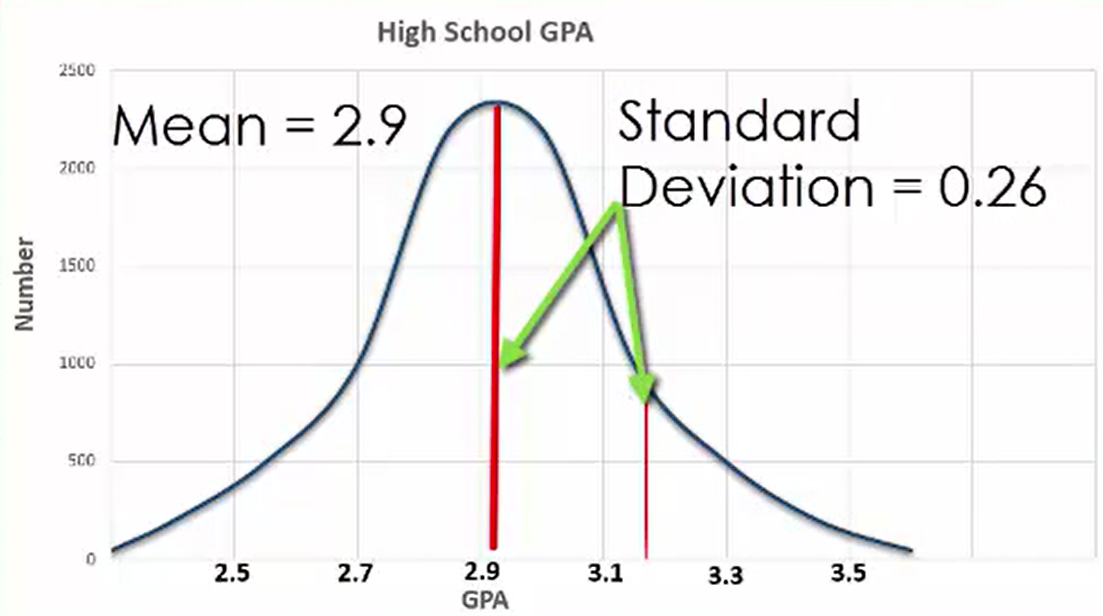term to know
Standard Deviation
Provides an indication of how close the data is distributed to the mean

## 2. Standard Deviation

By knowing the variability of a data set in terms of variance and standard deviation, you are generally able to determine what percentage of data falls within a certain range of values. You are better able to draw conclusions about your data when you have such information at your disposal. If you recall what a normal distribution is, you can see in the graph how the standard deviation illustrates the variability in the data set.

Looking at the an example of the miles per gallon of new cars, you can see that the standard deviation provides you with an indication of how close the data is distributed to the mean. You could encounter a situation where the data has a large standard deviation if you were to include large vehicles and trucks in your data set.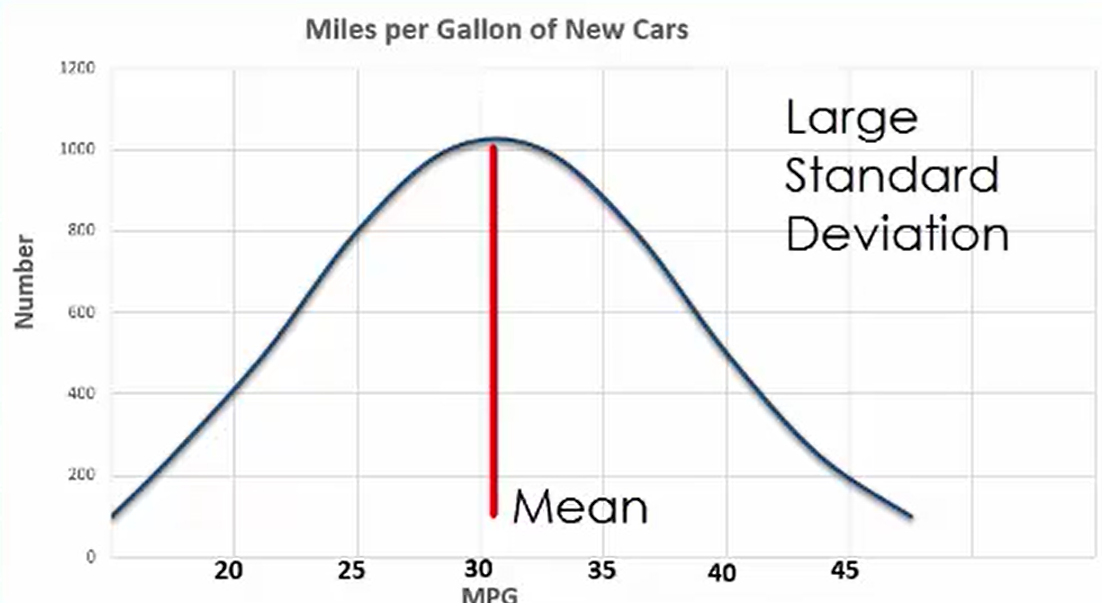On the other hand, if your data only consisted of small sedans, the standard deviation would likely be quite small. With a large standard deviation, the data will be spread out relatively far, whereas with a small standard deviation, the data would be much closer to the mean.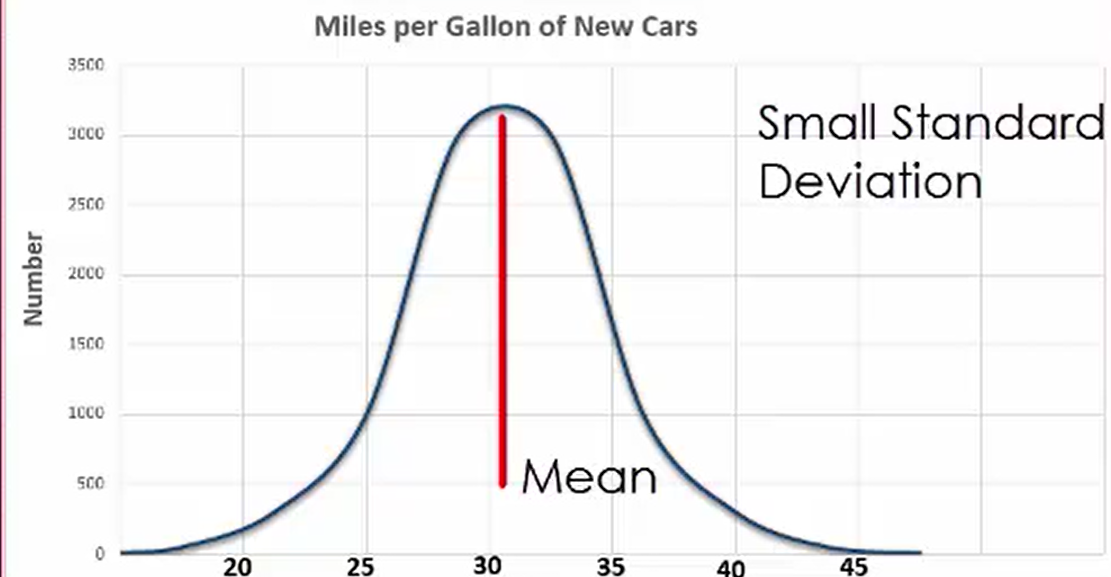term to know

Standard Deviation
Provides an indication of how close the data is distributed to the mean.

## 3. Variance

Much like standard deviation, variance also helps determine how spread out data is from the mean.

IN CONTEXT

Suppose that you conducted an experiment aimed at establishing the length of time that patients had to wait to see two different doctors. Both doctors had a mean wait time of 18 minutes, but the variation in the data was significantly different.

The standard deviation of the wait time for Dr. Smith would be 6 minutes, whereas for Dr. Jones it would be 2 minutes. If we were to only look at the mean, we would risk drawing poor conclusions regarding the validity of the experiment, especially considering that variance takes into account how widespread the data may be.

Generally speaking, a lower variance is preferable to a higher variance when trying to compare the results of two tests designed for learning more about a topic, as a lower variance indicates that the tests may validate one another.

Remember that the population is the entire group of people a researcher is interested in. It is typically very large. Therefore, it is much simpler to work with a sample instead, and use the sample to interpret information about the population.

The variance and a sample standard deviation can be found using the following steps:

First, compute the mean, x-bar, of the data set. Given a list of numbers, the mean is found by adding up all of the numbers and then dividing by how many numbers there are. This is expressed by the equation sum of x divided by n, where sum of x refers to the sum of all the numbers and n is how many numbers there are in the data set.

Next, subtract the mean from each number in the data set and square the difference. Now, add all of the values in the previous step. This quantity is called a sum of squares.

formula
Sum of Squares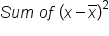Now we divide the sum of squares by n minus 1 (the total number of values there are in the data minus 1). The quantity we obtained is what we call the sample variance.

formula
Sample Variance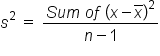Working through this on a calculator, you must first add all the numbers and then divide. When the variance comes from a sample, it is denoted by the term s squared. The standard deviation is then the square root of the variance, or s.

formula
Sample Standard Deviation (s)IN CONTEXT

This table shows the price of textbooks, and it includes eight different observations. The left column in red shows the total price of the textbooks for each individual book.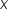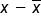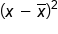145 -27 729
150 -22 484
165 -7 49
172 0 0
177 5 25
182 10 100
185 13 169
200 28 784

The first step in this case would be to determine what the sum of these values actually is. If you add them all up together we get 1,376. If you divide that by 8, you get a mean of 172.

The next step after that would be to subtract x-bar, which is 172, from the actual values of x. That is listed in the second column. Then square the difference. This is listed in the third column. Add them all up together, and you get a sum of squares of 2,340.

You can calculate the variance by taking that value and dividing it by n minus 1, or in this case 7. You get a variance of 334.28 for this sample. And in the last step to determine the standard deviation, take the square root of the sample variance, which in this case would be 18.28.

Sum of X = 1,376
Sum of X/n = 172
Sum of Squares = 2,340
Variance = 334.28
Standard Deviation = 18.28
term to know
Variance
Takes into account how widespread the data may be
summary
By determining how spread out data is from the mean, you can determine a measure of variability. There are two measures of variability. Standard deviation provides you with an indication of how close data is distributed to the mean. Variance takes into account how widespread data can be. It is found by taking the sum of squares.

Source: This work is adapted from Sophia author Dan Laub.

Terms to Know
Standard Deviation

Provides an indication of how close the data is distributed to the mean

Variance

Takes into account how widespread the data may be

Formulas to Know
Sample Standard DeviationSample VarianceSum of SquaresRating